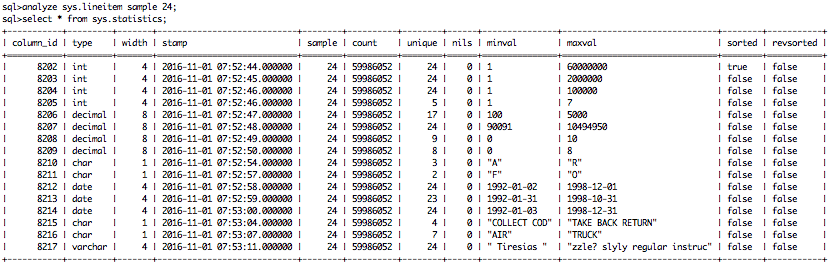# Table statistics

Statistics gathered over tables in the database can be found in the system table sys.statistics. This table is initially empty and explicitly filled or updated using the ANALYZE command:

 ANALYZE schemaname [ '.' tablename [ '('columnname , ...')' ] ]       [ SAMPLE size ]       [ MINMAX ]

You can gather statistics for a) all tables in a schema or b) all columns of a specific table or c) a list of specific columns of one table. You can only gather statistics of tables with real physical column data, so not for views.

Since statistics gathering involves accessing and profiling all table columns data, it can take considerable time, especially if the tables are large or you analyze all tables in a schema. You may consider to add an optional MINMAX which directs exclusion of the expensive unique count operation. Likewise, a SAMPLE size can be used to provide a quick, but imprecise impression.You can remove statistics data via SQL command: DELETE FROM sys.statistics. For example removing statistics for table: my_schema.my_table:

```DELETE FROM sys.statistics  WHERE column_id IN (SELECT c.id FROM sys.columns c  WHERE c.table_id IN (SELECT t.id FROM sys.tables t  WHERE t.schema_id IN (SELECT s.id FROM sys.schemas s  WHERE s.name = 'my_schema') AND t.name = 'my_table'));```